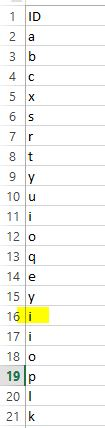# New to QlikView

Discussion board where members can get started with QlikView.

Highlighted
Contributor

## Set variable from the load table

Hello Users,

I am trying to set the variable from the excel table which is loaded.

The value of the variable is set based on the condition that it should pick the value of 0.75 of the total rows.

For example

Based on the attached excel sheet, value of the variable should be 'i' as it is the 0.75 of total rows from the excel (total rows=20, 0.75 of total row is 15(=20*.75=15),so 15th row of the excel sheet is 'i' from column ID.

Need to the set the variable value in load script not in chart.1 Solution

Accepted SolutionsMVP

## Re: Set variable from the load table

Try like:

Tab:

ID,

RowNo() as RowNum

FROM

[Variable.xlsx]

(ooxml, embedded labels, table is Sheet1);

Let vNoOfRows=NoOfRows('Tab');

Let v75RowNum=FieldValue('RowNum', vNoOfRows*.75);

Let v75Value=Lookup('ID','RowNum', v75RowNum);

2 RepliesMVP

## Re: Set variable from the load table

Try like:

Tab:

ID,

RowNo() as RowNum

FROM

[Variable.xlsx]

(ooxml, embedded labels, table is Sheet1);

Let vNoOfRows=NoOfRows('Tab');

Let v75RowNum=FieldValue('RowNum', vNoOfRows*.75);

Let v75Value=Lookup('ID','RowNum', v75RowNum);

Contributor III

## Re: Set variable from the load table

Hi,

Temp:

RowNo() as RowNumber

FROM

[Variable.xlsx]

(ooxml, embedded labels, table is Sheet1)

;

let vNofrows=NoOfRows('Temp')*0.75;

LET Row= peek('ID', \$(vNofrows)-1,'Temp');

Thanks,

Pooja